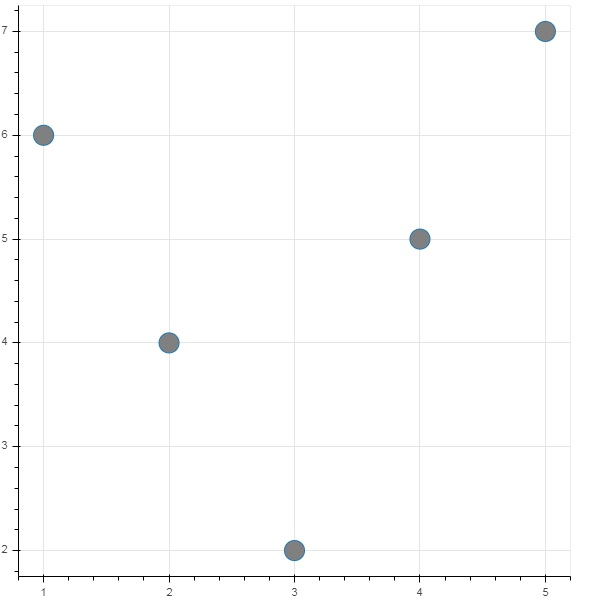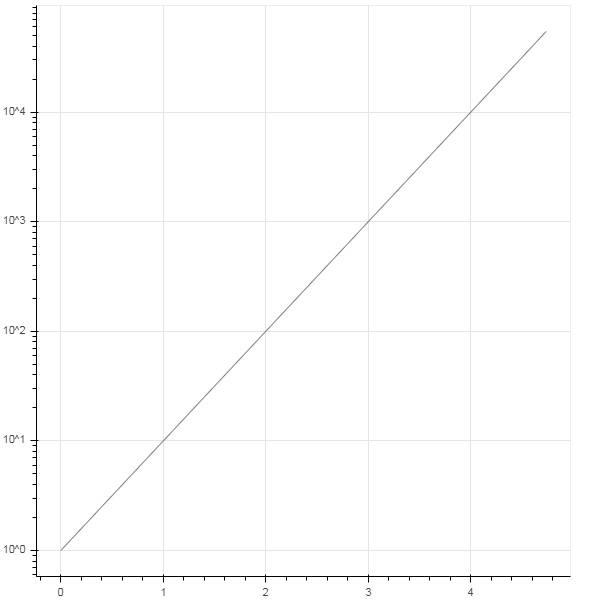# Bokeh - ColumnDataSource

Most of the plotting methods in Bokeh API are able to receive data source parameters through ColumnDatasource object. It makes sharing data between plots and ‘DataTables’.

A ColumnDatasource can be considered as a mapping between column name and list of data. A Python dict object with one or more string keys and lists or numpy arrays as values is passed to ColumnDataSource constructor.

### Example

Below is the example

```from bokeh.models import ColumnDataSource
data = {'x':[1, 4, 3, 2, 5],
'y':[6, 5, 2, 4, 7]}
cds = ColumnDataSource(data = data)
```

This object is then used as value of source property in a glyph method. Following code generates a scatter plot using ColumnDataSource.

```from bokeh.plotting import figure, output_file, show
from bokeh.models import ColumnDataSource
data = {'x':[1, 4, 3, 2, 5],
'y':[6, 5, 2, 4, 7]}
cds = ColumnDataSource(data = data)
fig = figure()
fig.scatter(x = 'x', y = 'y',source = cds, marker = "circle", size = 20, fill_color = "grey")
show(fig)
```

### OutputInstead of assigning a Python dictionary to ColumnDataSource, we can use a Pandas DataFrame for it.

Let us use ‘test.csv’ (used earlier in this section) to obtain a DataFrame and use it for getting ColumnDataSource and rendering line plot.

```from bokeh.plotting import figure, output_file, show
import pandas as pd
from bokeh.models import ColumnDataSource